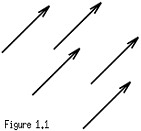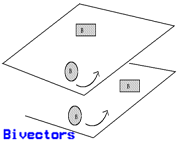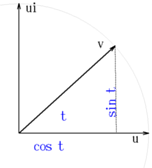# The Geometric Algebra of 3D Euclidean SpaceThe Rules of the Game
 We introduce here a collection of symbols and some rules for their manipulation. In this way we build a language that will prove tremendously useful for studying nature once a geometric interpretation is attached to the otherwise abstract symbols. Let the symbols e1, e2, e3 denote three arbitrary objects for which we shall define an associative operation of multiplication among themselves. Consider now the set of all the products of the original three objects (at this point this could be an infinite set containing all the possible sequences of products). In this new set introduce the operations of addition and multiplication by a number with the usual properties for the "+" and scalar multiplication that are required for a linear (vector) space. We call the set of objects generated in this way (i.e. all linear combinations of all products of the basic three objects e1,e2, e3) the geometric algebra of euclidean 3 dimensional space iff the operation of multiplication is distributive with respect to the sum and the basic vectors square to 1 and anticommute with each other. More explicitly we require: e1 e1 = 1 e2 e2 = 1 e3 e3 = 1 e1 e2 = - e2 e1 e1 e3 = - e3 e1 e2 e3 = - e2 e3 e1 e2 e3 = (e1 e2) e3 = e1 (e2 e3) For reasons that will be evident later we shall agree to call the objects e1, e2, e3 and all the linear combinations of them, vectors. Notice that by taking products of the basic objects we get out of the space generated by them. In particular we generate the unit scalar and we shall prove that also two new kinds of objects different from scalars and vectors are obtained. Objects of the type e1 e2 will be called bivectors and the ones of the type e1 e2 e3 will be called trivectors for obvious reasons. Notice also that there are no more types besides scalars (0-vectors), vectors (1-vectors), bivectors (2-vectors) and trivectors (3-vectors). All other products like, e3 e2 e3 e1 e3 e3 e2 e1 simplify to one of the basic types 0, 1, 2, or 3-vectors by the rules stated above: associativity, square 1 and anticommutativity. For example the above long product is nothing but another name for the unit scalar 1 a 0-vector. Let us investigate some immediate consequences of the previous assumptions. If a is a vector i.e. a = x e1 + y e2 + z e3 then by the rules above: a a = ( x e1 + y e2 + z e3) (x e1 + y e2 + z e3) = x^2 + y^2 + z^2 = innerprod(a,a) and we have that the square of every vector is the same as the positive scalar equal to the square of its length. In particular if we add two vectors a and b we obtain another vector (a + b) whose square is: (a + b) (a + b) = a^2 + b^2 + ab + ba and as we know, the square of every vector equals the innerprod of the vector with itself we obtain, using the linearity of innerproducts, that, a^2 + b^2 + 2 innerprod(a,b) = a^2 + b^2 + ab + ba From where we deduce that: innerprod(a,b) = ( ab + ba ) / 2 So if we didn't know about the innerproduct we could have defined it as above, as the symetrized geometric product of two vectors. Clearly, ab = ( ab + ba ) / 2 + ( ab - ba ) / 2 The first term is the innerproduct and the second, which is the anti-symetrized product of a with b is an object of the type "bivector". We call this new product the outer or wedge product of a with b. Thus, ab = innerprod(a,b) + wedge(a,b) Notice that as a special case we have: e1 e2 = wedge(e1,e2) = e12 The last equality is just the definition of a new notation that allows me to save typing. We use similar notations for the other outer products of basis elements: e23, e31, e32.. etc. Now that it should be clear that e1 is a vector we drop the bold-face type and we simply write e1, e2, e3. Here is our first proposition. Proposition The set { 1, e1, e2, e3, e23, e31, e12, e123 } is a basis for the linear (vector) space generated by taking all the possible geometric products of the vectors e1,e2,e3, and all the linear combinations of these products. Thus, every element in the geometric algebra has a unique expression as a linear combination of the elements in this basis, A = u0 + u1 e1 + u2 e2 + u3 e3 + v1 e23 + v2 e31 + v3 e12 + v0 e123 where A is an arbitrary element of the algebra (we call it a multivector) and the coefficients u0, u1,...,v3,v0 are scalars. proof We only need to show that the 8 elements of the set are linearly independent for we already know that they span the algebra. So let us take a general multivector A = 0 and show that this is only possible when all the scalar coefficients u0,...,v0 are 0. To show this, first notice that by taking linear combinations of vectors we always get another vector or perhaps the scalar 0, but never a bivector or a trivector. The same is true for scalars, bivectors and trivectors. By multiplying an object of one type by a scalar and adding this to an object of the same type we can get either the scalar 0 or another object of the same type. Objects of different grades don't mix by taking linear combinations. We say that the geometric algebra is a "graded algebra". Hence, we need only to prove that the 3 vectors are l.i. and that the three bivectors are l.i. If u1 e1 + u2 e2 + u3 e3 = 0 then by multiplying through on the left by e1 we get, u1 + u2 e12 - u3 e31 = 0 and since the bivectors don't mix with the scalars the only way a (scalar) + (bivector) = 0 is that u1 = 0 and u2 e12 - u3 e31 = 0 had we multiplied by e2 or e3 we would have obtained u2 =0 or u3 = 0. So if the three vectors e1, e2, e3 square to 1 and anticommute they must be l.i. In a similar way we show that the three bivectors, e23, e31, e12 are l.i. From v1 e23 + v2 e31 + v3 e12 = 0 we can deduce that v1 = v2 = v3 = 0. Multiplying through on the left by e32, we obtain v1 e32 e23 + v2 e32 e31 + v3 e32 e12 = 0 but e32 e23 = e3 e2 e2 e3 = e3 (e2 e2) e3 = e3 (1) e3 = e3 e3 = 1. Also e32 e31 = - e12, and e32 e12 = e31 so the above equation becomes, v1 - v2 e12 + v3 e31 = 0 from where we deduce that v1 = 0. If instead of multiplying by e32 we multiply by e13 we get v2 = 0, and multiplying by e21 we obtain v3 = 0. We conclude that the three bivectors are in fact l.i. and the whole geometric algebra as a linear space has dimension: 1 + 3 + 3 + 1 = choose(3,0) + choose(3,1) + choose(3,2) + choose(3,3) = 2^3 = 8. Yes but what are these bivectors and trivectors? To answer this question we need to provide the geometric interpretation associated with the algebra. The short answer to the question: What is a bivector? is: a new kind of number.Let us start with vectors. A vector, is a directed number. A number consisting of two parts: an amount, and a direction. It can be visualized as an arrow (see Figure 1.1). The length of the arrow represents the amount and the direction is just the direction of the arrow. Vectors are pieces of oriented lines. There is a sense of going forward on the line and backwards on the line. Parallel arrows of the same length and with the same orientation are represented by the same vector no matter where the arrow is located.
 The axioms for a vector space are designed to encode the intuitive idea of addition of arrows and multiplication of an arrow by a number. Thus, we can encode statements like: Moving 3 miles East and after that moving 4 miles North takes me to the same place as Moving 5 miles straight approximately NE can be encoded concisely and precisely by: 3 e1 + 4 e2 = 5 ( (3/5) e1 + (4/5) e2 )This is why the algebra of vectors is so useful for describing displacements, and forces and many other physical quantities. Similarly with bivectors. Bivectors are directed numbers in two dimensions. They also have two parts an amount and a direction. The amount is a two-dimensional amount (an area) and the direction is a two-dimensional direction the direction of a plane. Vectors are pieces of oriented lines, bivectors are pieces of oriented planes. There is a sense of going forward (covering more area) on the plane and a sense of going backwards (covering less area) on the plane. You may think of it as unrolling a roll of cloth or rolling it back. Two pieces with the same area on two parallel planes with the same orientation are represented by the same bivector no matter what the shape of the area looks like or where the planes are located.
 By now you could guess what a trivector is. A trivector is a three dimensional number. It is a piece of 3 dimensional space. The 3 dimensional amount is the volume in the piece and the direction is the direction of the 3 space the piece is in. Again the space is oriented so that there is a sense of unrolling and rolling space that corresponds to positive and negative volumes. Analogously for 4,5,... r-vectors that are needed in dimensions higher than 3. The Two Dual Interpretations of r-Numbers Every time we know how to multiply symbols we can assign to each symbol in the space, in addition to what it labels, another interpretation as an operator. A real number for example, the number 2 say, labels an amount of 2 but also encodes the operator "doubler" or "multiplicator by 2". Same thing happens in matrix algebra. Each matrix encodes a system of linear equations and at the same time a transformation of a system of linear equations. The objects in the geometric algebra are particularly useful for representing the isometries of the space. The isometries are those transformations of the space that preserve the distance between two points. In euclidean space these are, translations, rotations and reflections and they are all linear. We shall study linear transformations and isometries in detail later on. For the moment let us investigate the action of multiplying by e12 any multivector generated by multiplications and linear combinations of e1 and e2 only. We have: e1 e12 = e1 (e1 e2) = (e1 e1) e2 = 1 e2 = e2 e2 e12 = e2 (e1 e2) = - e2 (e2 e1) = -(e2 e2) e1 = - e1 e12 e12 = - e12 e21 = -1 u0 e12 = e12 u0 we can see that multiplication by e12 on the right transforms e1 into e2, and e2 into -e1, in other words, e12 rotates e1 and e2 in 90 degrees counter- clockwise (see fig below). The fact that e12 squares to -1 only encodes the simple fact that rotating in 90 degrees two times in a row is the same as rotating in 180 degrees which is equivalent to reverse orientation. Thus if u = u1 e1 + u2 e2 is an arbitrary vector on the plane generated by e1 and e2 we have: u e12 = u1 e2 - u2 e1 which is of course the rotation of u in 90 degrees counter-clockwise. Notice that denoting the innerproduct with a simple dot: u . (u e12) = u1^2 e1.e2 - u1 u2 e1^2 + u1 u2 e2^2 - u2^2 e2.e1 and simplifies to 0 by the multiplication rules of the basic vectors. It is convenient to denote by i the multivector of highest degree of size 1. Thus, if we are constrained to the plane generated by e1 and e2, the unit bivector on this plane is i = e12. All other multivectors of the highest degree are scalar multiples of i and for this reason i is also known as the pseudoscalar of the space. In dimension 3, i = e123, etc... it should always be clear, from the context, in what space we are working and what is the i there.The unit vectors e1 and e2 are orthogonal. The angle measured from e1 to e2 is 90 degrees (usually measured counter-clockwise). The multiplication by (e1 e2) on the right of a vector rotates the vector 90 degrees in the same direction that e1 goes towards e2 (usually counter-clockwise). Let us now see what happens when we post-multiply by the product of two unit vectors u v that are now separated by an arbitrary angle t. In other words let u and v be vectors with u^2 = v^2 = 1
 Now (see fig) the innerproduct u.v = cos t. Their wedge product will be proportional to the unit pseudoscalar of the plane generated by u and v. We have (see fig) that, uWv = s i = (sin t) i. Thus, (see fig) e1 (uv) = e1 ( cos t + i sin t) = (cos t) e1 + (sin t) e2 e2 (uv) = e2 ( cos t + i sin t) = (cos t) e2 - (sin t) e1 u (uv) = u^2 v = v we have found then, that post-multiplication by uv is the operation of rotation on the plane containing u and v in an angle equal to the angle between these two unit vectors and in the direction from u towards v. All the information about a rotation: the plane, the angle and the direction (i.e. clockwise or counter-clockwise) is encoded very efficiently in the operation of multiplication by uv. There is no easier way to represent rotations.

[Formulas] [Linear Functions]
Carlos Rodriguez <carlos@math.albany.edu>
Last modified: Tue Apr 24 08:48:11 EDT 2001